# Cardinality of Sets | Word Problems based on Sets and Venn Diagrams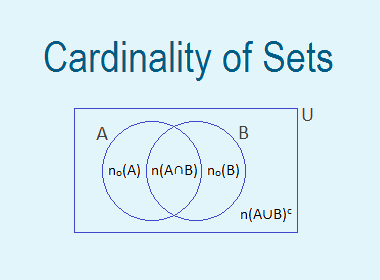Cardinality of Sets

Let, A be a finite set. The number of elements in set A is called the cardinal number of set A. It is denoted by n(A)If A = {a, b, c, d, e}, the number of elements in set A is 5, therefore the cardinal number of set A, i.e. n(A) = 5.

********************

10 Math Problems officially announces the release of Quick Math Solver, an Android App on the Google Play Store for students around the world.

********************

Let, U be the universal set, A and B be any two finite and overlapping subsets of U. Then,n(U) = Number of elements in set U

n(A) = Number of elements in set A

n(B) = Number of elements in set B

n(AB) = Number of elements in set AB

n(A∩B) = Number of elements in A∩B

no(A) = Number of elements in set A only

no(B) = Number of elements in set B only

n(AB)c = Number of elements in set (AB)c

The properties based on the cardinal number of sets are called the cardinality of sets.

Method for finding cardinality of sets

Look at the following Venn-diagrams,In above venn-diagram, sets A and B are disjoint sets with n(A) = 4 and n(B) = 3. Both the sets A and B doesn’t have any common elements. So, n(A∩B) = 0 and n(AB) = 4 + 3 = 7.

Therefore, for disjoint sets A and B,

n(AB) = n(A) + n(B)

n(A∩B) = 0

Again, look at the following Venn-diagram,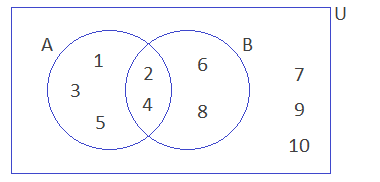In above venn-diagram, sets A and B are intersecting or overlapping sets with n(A) = 5 and b(B) = 4. Sets A and B have 2 elements common. So, n(A∩B) = 2. There are altogether 7 elements within A and B. So, n(AB) = 7. Which can be obtained by applying the formula,

n(AB) = n(A) + b(B) – n(A∩B)

= 5 + 4 – 2

= 7

The total elements within the universal set U is 10. So, n(U) = 10. Outside of A and B, there are 3 elements. So, n(AB)c = 3. So, we can have the following additional formula,

n(AB) = n(U) – n(AB)c

n(AB)c = n(U) – n(AB)

n(U) = n(AB) + n(AB)c

There are 3 elements in A – B or A only part, so no(A) = 3. Similarly no(B) = 2. So, we have the following results as well,

no(A) = n(A) – n(A∩B)

no(B) = n(B) – n(A∩B)

n(AB) = no(A) + no(B) + n(A∩B)

n(U) = no(A) + no(B) + n(A∩B) + n(AB)c

Therefore, for overlapping sets A and B,

n(AB) = n(A) + b(B) – n(A∩B)

n(AB) = n(U) – n(AB)c

So, we have the following list of cardinality formula of sets:

If A and B are subsets of universal set U then,

1.     n(AB) = n(A) + n(B) – n(A∩B)

2.     n(A∩B) = n(A) + n(B) - n(AB)

3.     n(AB)c = n(U) – n(AB)

4.     no(A) = n(A) – n(A∩B)

5.     no(B) = n(B) – n(A∩B)

6.     n(AB) = no(A) + no(B) + n(A∩B)

7.     n(U) = no(A) + no(B) + n(A∩B) + n(AB)c

8.     n(U) = n(A) + n(B) + n(AB)c – n(A∩B)

Some languages and their set-notations used in the problems on cardinality of  sets:

1.     Both = n(A∩B)

2.     At least one = n(AB)

3.     Exactly one = no(A) + no(B)

4.     None of them = n(AB)c

Word Problems based on Sets and Venn Diagrams

Worked Out Examples

Example 1: In the given venn-diagram A and B are two subsets of an universal set where n(U) = 50, n(A) = 23, n(B) = 20 and n(A∩B) = 8.Find the following:

a.     n(AB)

b.     n(AB)c

c.     no(A)

d.     no(B)

e.     Exactly one =?

Solution:

Here,

n(U) = 50

n(A) = 23

n(B) = 20

n(A∩B) = 8

a.     n(AB) = n(A) + n(B) – n(A∩B)

= 23 + 20 – 8

= 35

b.     n(AB)c = n(U) – n(AB)

= 50 – 35

= 15

c.     no(A) = n(A) – n(A∩B)

= 23 – 8

= 15

d.     no(B) = n(B) – n(A∩B)

= 20 – 8

= 12

e.     Exactly one = no(A) + no(B)

= 15 + 12

= 27

Example 2: Out of 50 students of class IX, 25 students liked to play football, 35 liked to play basketball and 15 liked to play both the games. How many students do not like to play any games? Show the above information in a Venn-diagram.

Solution:

Let the set of students who like football and basketball be F and B.

n(U) = 50

n(F) = 25

n(B) = 35

n(F∩B) = 15

n(FB)c = ?

We Know,

n(FB) = n(F) + n(B) – n(F∩B)

= 25 + 35 – 15

= 45

Now,

n(FB)c = n(U) – n(FB)

= 50 – 45

= 5

Venn-diagram,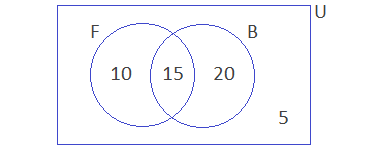5 students do not like to play any games. Ans.

Example 3: In a class of 30 students, 20 students like to play cricket and 15 like to play volleyball. Also, each student like to play at least one of the two games. How many students like to play both games? Illustrate the above information in a Venn-diagram.

Solution:

Let, the set of students who like to play cricket and volleyball be C and V.

n(U) = 30

n(C) = 20

n(V) = 15

Each student like to play at least one game. Therefore,

n(CV) = n(U) = 30

Now,

n(C∩V) = n(C) + n(V) – n(CV)

= 20 + 15 – 30

= 5

Venn-diagram,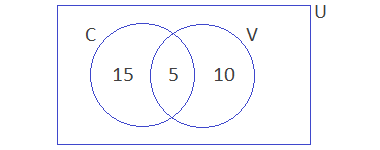5 students like to play both games. Ans.

Example 4: In a survey of a community, it was found that 85% of people like the winter season and 65% like the summer season. If none of them did not like both seasons,

a.     Present the above information in a Venn-diagram.

b.     What percent liked both the seasons?

c.     What percent liked winter season only?

Solution:

Let, the set of people who liked winter and summer be W and S.

n(U) = 100%

n(W) = 85%

n(S) = 65%

Since none of them did not like both the seasons,

n(WS) = n(U) = 100%

Now,

n(W∩S) = n(W) + n(S) – n(WS)

= 85 + 65 – 100%

= 50%

a.     Venn-diagram,b.     n(W∩S) = 50% like both the seasons.

c.     no(W) = n(W) – n(W∩S)

= 85 – 50%

= 35% like winter season only.

Example 5: In a group of 100 people, 72 can speak English and 43 can speak Hindi. How many can speak English only? How many can speak Hindi only and how many can speak both Hindi and English?

Solution:

Let the set of students who can speak English and Hindi be E and H.

n(U) = 100

n(E) = 72

n(H) = 43

Since, each person can speak at least one language,

n(EH) = n(U) = 100

Now,

n(E∩H) = n(E) + n(H) – n(EH)

= 72 + 43 – 100

= 15

Now,

no(E) = n(E) – n(E∩H) = 72 – 15 = 57

no(H) = n(H) – n(E∩H) = 43 – 15 = 28

Therefore,

no(E) = 57 people can speak English only.

no(H) = 28 people can speak Hindi only.

n(E∩H) = 15 people can speak both the languages.

Example 6: Out of 100 students, 80 passed science, 71 mathematics, 10 failed both subjects and 7 did not appear in the examination. Find the number of students who passed both subjects by representing the above information in a Venn-diagram.

Solution:

Let the set of students who passed science and mathematics be S and M.

n(U) = 100

n(S) = 80

n(M) = 71

n(SM)c = 10 + 7 = 17

n(S∩M) = ?

We Know,

n(SM) = n(U) – n(SM)c

= 100 – 17

= 83

Now,

n(S∩M) = n(S) + n(M) – n(SM)

= 80 + 71 – 83

= 68

Venn-diagram,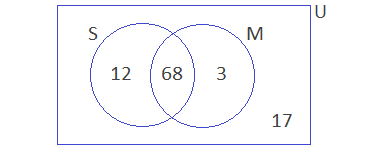Hence 68 students passed both the subjects.

Example 7: In a survey of 100 farmers, the ratio of the farmers who grew paddy and wheat is 2:3. If 10 farmers grew both and 20 grew none of them, find the numbers of farmers who grew paddy only by using Venn-diagram.

Solution:

Let, the set of farmers who grew paddy and wheat be P and W.

n(U) = 100

n(P) = 2x, and n(W) = 3x (let)

n(P∩W) = 10

n(PW)c = 20

We know,

n(PW) = n(U) – n(PW)c

= 100 – 20

= 80

i.e.     n(P) + n(W) – n(P∩W) = 80

or,     2x + 3x – 10 = 80

or,     5x = 80 + 10

or,     5x = 90

or,     x = 90/5

or,     x = 18

Therefore,

n(P) = 2x = 2 × 18 = 36

n(W) = 3x = 3 × 18 = 54

And,

no(P) = n(P) – n(P∩W)

= 36 – 10

= 26 Ans.

Venn-diagram,Example 8: In a group of total students 35 enrolled in art class and 57 enrolled in dance class. Find the number of students who are either in art class or in dance class, if the situation is:

a.     When two classes meet at the same hour.

b.     When two classes meet at a different hour and 12 students are enrolled in both activities.

Solution:

Let, the set of students enrolled in art and dance class be A and D.

n(A) = 35

n(D) = 57

a.

When two classes meet at the same hours then n(A∩D) = 0.

n(AD) = n(A) + n(D) – n(A∩)

= 35 + 57 – 0

= 92

92 students are either in art or in dance class.

b.

When two classes meet at different hours then n(A∩D) = 12.

n(AD) = n(A) + n(D) – n(A∩)

= 35 + 57 – 12

= 80

80 students are either in art or in dance class.

Example 9: In a survey, it was found that 80% of people liked oranges, 85% liked mangoes and 75% liked both. But 45 people liked none of them. By drawing a Venn diagram, find the number of people who were in the survey.

Solution:

Let the set of students who like orange and mango be O and M.

n(U) = 100%

n(O) = 80%

n(M) = 85%

n(O∩M) = 75%

n(OM)c = 45

We Know,

n(OM) = n(O) + n(M) – n(O∩M)

= 80% + 85% – 75%

= 90%

Now,

n(OM)c = n(U) – n(OM)

= 100% – 90%

= 10%

Let, the total number of people in the survey be ‘x’.

10% of x = 45

or,     10/100 × x = 45

or,     1/10 × x = 45

or,     x = 450

Venn-diagram,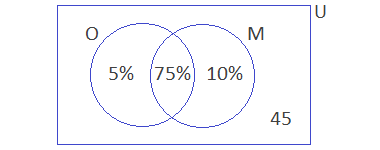Hence, the number of people who were in the survey is 450.

Example 10: In an election, there were 400 voters, A and B were two candidates. The ratio of votes of A and B is 4:3. If 40 voters cast votes for both and 10 voters didn’t cast the vote, find

a.     No. of votes got by A.

b.     Total no of valid votes.

Solution:

Here,

n(U) = 400

The ratio of 4:3 is only for the valid votes, therefore

no(A) = 4x and no(B) = 3x (let)

n(AB) = 10

We know,

no(A) + no(B) + n(A∩B) + n(AB)c = n(U)

i.e.     4x + 3x + 40 + 10 = 400

or,     7x + 50 = 400

or,     7x = 400 – 50

or,     7x = 350

or,     x = 350/7

or,     x = 50

Therefore,

a.     No of votes got by A = no(A) = 4x = 4 × 50 = 200

b.     Total no. of valid votes = no(A) + no(B) = 4x + 3x = 7x = 7 × 50 = 350

If you have any questions or problems regarding the Cardinality of Sets, you can ask here, in the comment section below.